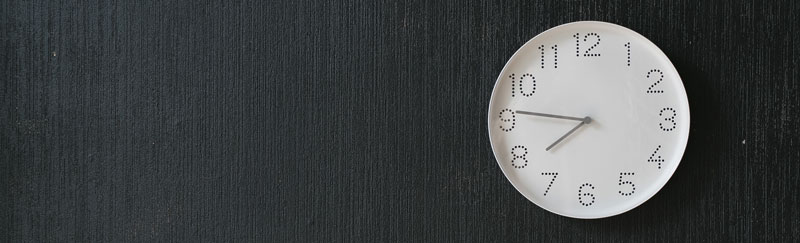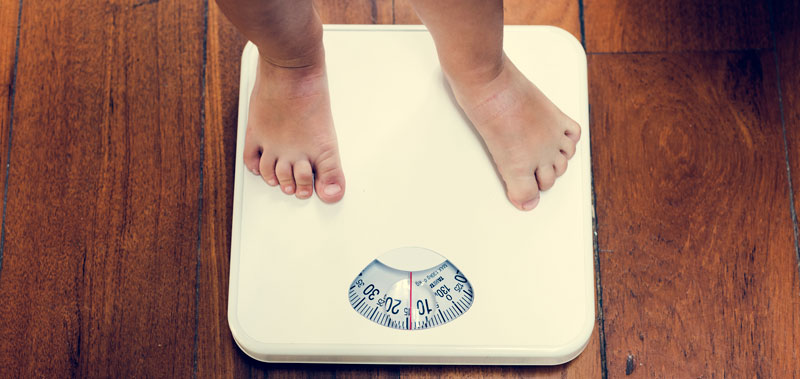# What are the Units of Measurement – An Introductory Lesson

## What is measurement

Measurement is basically to find a number that represents something. The simple English definition of measurement is; the act or process of measuring different units such as; size, length, or amount.

## Significance of learning about measurement?

We use measurement in our daily lives, all the time. While deciding how many teaspoons of sugar to add while preparing three cups of tea, you need to estimate the quantity of sugar required. To measure the time it could take for you to drive down to the airport from home, one measures the distance and guesstimate the time of arrival.

The sooner children learn about measurement, the better it is for them. Preschoolers start by measuring weight which is the simplest to understand and then gradually learn to use a ruler & graph charts to analyze complex data in higher standards.

## Different standard measurement units are

• Length
• Time
• Weight## Length

Length is measuring an object or distance from one point to another. Length tells us how long an object is, or how far the distance is between two points. Length is used to measure the size of the object and the distance between points.

### Common units for measuring length

The standard unit of measuring length is Meter represented as ‘m’. For bigger measurements, the unit changes to ‘kilometers’. For smaller measurements, the same can be represented with; centimeter, millimeter, and decimeter.

#### Millimeter (mm)

Millimeters are used to measure very minuscule or tiny lengths. Imagine a sharp pencil point. The tip of the pencil would be around (1 mm).

#### Centimeter (cm)

A centimeter is used to measure small objects. Size of a tiny square paper, a key, a pen, etc. can be measured best using the centimeters scale. A standard metric ruler is 30 centimeters long.

#### Meter (m)

A meter is used to measure big lengths. A classroom can be measured in terms of meters. Table tops and window frames, can all be equated to the measurement of meter. 1 m = 100 cms

#### Kilometer (km)

Very long distances are measured in kilometers. The distance from your house to the mall or from distance from one city to another is measured using kilometers. 1 km = 1000 m

Other units for measuring length are; inch, foot, yard, and mile.

Give children objects of different lengths and ask students to measure and compare them. A ruler is thus introduced and children learn how to read the cm and inch markings.

Now ask children to measure their own waist area, using a measuring tape. Take the class outdoors into the garden and ask them to measure the length by holding the meter stick or a retractable measuring tape from one end of the lawn to the other. This helps them calculate meters and then covet meter to an inch or meter to a centimeter.

To teach children about the significance of 1 kilometer, take them for a drive and download any of the distance calculating apps on your phone. At every one-kilometer mark, announce that we have travelled that much of a distance, and then total it up to determine how far has the car actually travelled. Also, help them convert kilometer to meter.

## Time

Time helps us prepare a schedule, set a date for an event, and conduct our daily activities as per plan. Introduce children to a clock and teach them about the second hand, minute hand, and hour hand. Start by simple time readings such as; 5:30 and 2:15, and then go to more complex readings such as; 9:53 or 13:45 etc.Take an English calendar and point to the boxes representing different numbers. Explain the concept of date and how each month is made of 30 or 31 days, with February being an exception having 28 or 29 days. Turn the pages over 12 times to run them through the months January to December and the days of the week subsequently.

• Seconds (s)
• Minutes (min)
• Hours (hr)
• Day
• Week
• Month
• Year

• 60 s = 1 min
• 1 hours = 60 min
• 1 day = 24 hours
• 1 week = 7 days
• 1 month = 30 or 31 days (February has 28 or 29 days)
• 1 year= 12 months

## Weight

Weight is the measure of the force of gravity acting on a body. It is the amount of matter an object or thing consists.

#### Milligram (mg)

It is used to measure very light objects. Imagine the weight of a light feather or a dose of medication, these are very insignificant in weight and thus use the unit of a milligram.

#### Gram (g)

A gram is used to measure weights of objects that are slightly more significant. It’s a common terminology in households and hotel kitchens because every now and then one uses this measurement to know the weight of ingredients such as; pasta, sugar, flour, butter, and veggies while preparing a dish.

1 g = 1000 mg

#### Kilogram (kg)

Most of our body weights are measured in kilograms. Even things like a vehicle’s weight, or the weight of a trolley bag at the airport, are measured in kilograms.

1 kg = 1000 g

Introduce students to a balancing scale and place objects of different weights on either side of its arm. Observe which arm goes down and which remains on the top. In this way compare heavy and light, or heavy and heavier, or light and lighter, objects.To learn about the different units of measurement, Mathseeds devices fun activities, lessons, and puzzles for children between 3-9 years of age and guides them through their learning journey. Mathseeds is a popular subject-specific platform renowned in the Middle East, UK, US, and Australia. Educators of K-12 schools all over UAE in Dubai & GCC, encourage parents to use Mathseeds to reinforce concepts, as an after-class additional Math practice session for kids.